# md5(unix)原理分析

## Magic

magic是表明这一段哈希是通过什么算法得到的，对应关系如下：

```\$0 = DES
\$1 = MD5
\$2a(2y) = Blowfish
\$5 = SHA-256
\$6 = SHA-512
```

`\$5\$DnnkiE71Scb5\$lHT.SBfgQKoiTi8cF.cbuxlZ9ZBVFG8CGDxh8CpgPe8`

`\$6\$I7iRFjXdW9rZA2\$/4WJ35KCqtrfc3BFmoargIm8WiKhY5cSBuJIb7ItjO0I7Dj99ZVIPZ3fgKvxaDgZqrWNWwL5aSVwQUkd8D7LT0`

```php -r "echo strlen(base64_decode('Hsrx102ek28d03B5dqgAv/'));"
```## Salt

salt是此次哈希的盐值，长度是8位，超过8的后面的位数将不影响哈希的结果。

`\$1\$Dx1bONFt\$Hsrx102ek28d03B5dqgAv/`

`1ecaf1d74d9e936f1dd3707976a800bf:Dx1bONFt`

```<?php
namespace Md5Crypt;
class Md5Crypt
{
static public \$itoa64 = './0123456789ABCDEFGHIJKLMNOPQRSTUVWXYZabcdefghijklmnopqrstuvwxyz';
// [a-zA-Z0-9./]

static protected function to64(\$v, \$n)
{
\$itoa64 = self::\$itoa64;
\$ret = '';

while(--\$n >= 0) {
\$ret .= \$itoa64{\$v & 0x3f};
\$v = \$v >> 6;
}
return \$ret;
}

static public function apache(\$pw, \$salt = NULL)
{
\$Magic = '\$apr1\$';

return self::unix(\$pw, \$salt, \$Magic);
}

static public function unix(\$pw, \$salt = NULL, \$Magic = '\$1\$')
{
\$itoa64 = self::\$itoa64;

if(\$salt !== NULL) {
// Take care of the magic string if present
if(substr(\$salt, 0, strlen(\$Magic)) == \$Magic) {
\$salt = substr(\$salt, strlen(\$Magic), strlen(\$salt));
}
// Salt can have up to 8 characters
\$parts = explode('\$', \$salt, 1);
\$salt = substr(\$parts, 0, 8);
} else {
\$salt = '';
mt_srand((double)(microtime() * 10000000));

while(strlen(\$salt) < 8) {
\$salt .= \$itoa64{mt_rand(0, strlen(\$itoa64)-1)};
}
}

\$ctx = \$pw . \$Magic . \$salt;

\$final = pack('H*', md5(\$pw . \$salt . \$pw));

for (\$pl = strlen(\$pw); \$pl > 0; \$pl -= 16) {
\$ctx .= substr(\$final, 0, (\$pl > 16) ? 16 : \$pl);
}

// Now the 'weird' xform
for(\$i = strlen(\$pw); \$i; \$i >>= 1) {
if(\$i & 1) {                // This comes from the original version,
\$ctx .= pack("C", 0);   // where a memset() is done to \$final
} else {                    // before this loop
\$ctx .= \$pw{0};
}
}

\$final = pack('H*', md5(\$ctx)); // The following is supposed to make
// things run slower

for(\$i = 0; \$i < 1000; \$i++) {
\$ctx1 = '';
if(\$i & 1) {
\$ctx1 .= \$pw;
} else {
\$ctx1 .= substr(\$final, 0, 16);
}
if(\$i % 3) {
\$ctx1 .= \$salt;
}
if(\$i % 7) {
\$ctx1 .= \$pw;
}
if(\$i & 1) {
\$ctx1 .= substr(\$final, 0, 16);
} else {
\$ctx1 .= \$pw;
}
\$final = pack('H*', md5(\$ctx1));
}

// Final xform
\$passwd = '';
\$passwd .= self::to64((intval(ord(\$final{0})) << 16)
|(intval(ord(\$final{6})) << 8)
|(intval(ord(\$final{12}))),4);
\$passwd .= self::to64((intval(ord(\$final{1})) << 16)
|(intval(ord(\$final{7})) << 8)
|(intval(ord(\$final{13}))), 4);
\$passwd .= self::to64((intval(ord(\$final{2})) << 16)
|(intval(ord(\$final{8})) << 8)
|(intval(ord(\$final{14}))), 4);
\$passwd .= self::to64((intval(ord(\$final{3})) << 16)
|(intval(ord(\$final{9})) << 8)
|(intval(ord(\$final{15}))), 4);
\$passwd .= self::to64((intval(ord(\$final{4}) << 16)
|(intval(ord(\$final{10})) << 8)
|(intval(ord(\$final{5})))), 4);
\$passwd .= self::to64((intval(ord(\$final{11}))), 2);

// Return the final string
return \$Magic . \$salt . '\$' . \$passwd;
}
}
```

```<?php
include_once("php-crypt-md5/library/Md5Crypt/Md5Crypt.php");

\$salt = "Dx1bONFt";

```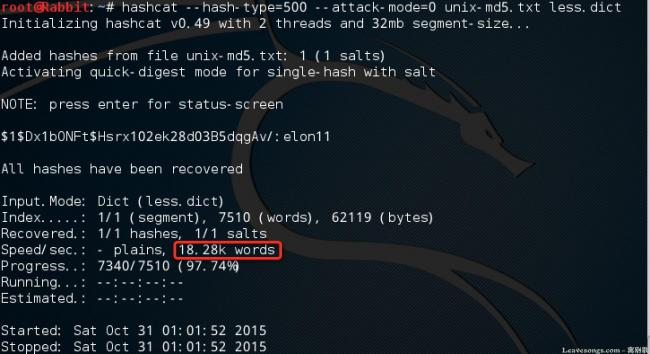7510个字典，仅用1秒不到跑完，速度为18.28k/s。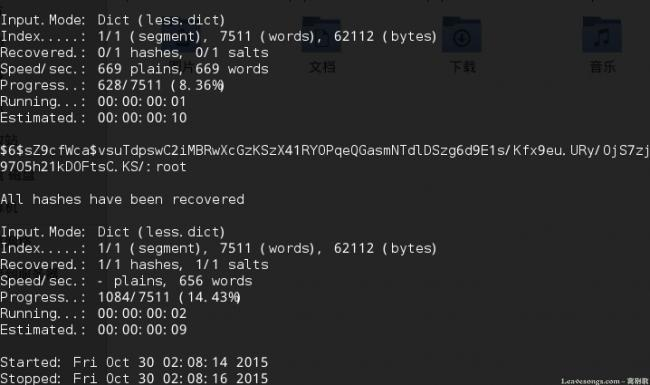```hashcat --hash-type=3200 --attack-mode=0 joomla.txt less.dict
```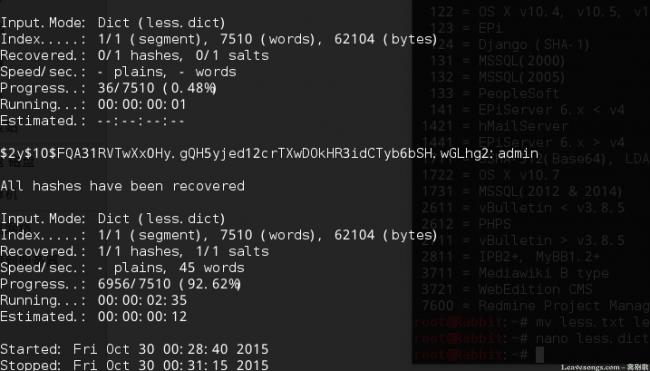cost在Blowfish算法中就是延缓其速度，增加破解难度的选项，如果将cost设置为12，生成的hash，破解起来速度可以降到10 words/s: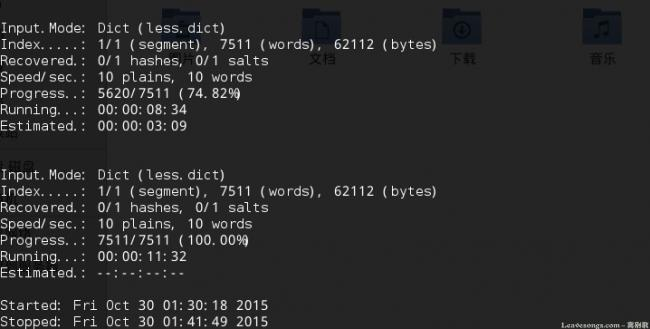## 开发与渗透中如何生成hash

```echo crypt("admin", '\$1\$12345678');
```

```echo crypt("admin", '\$6\$12345678');
```

```echo password_hash("123123", CRYPT_BLOWFISH);
```

```echo password_hash("123123", CRYPT_BLOWFISH, ["cost" => 12]);
```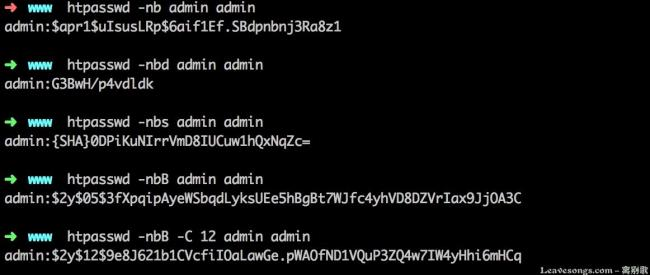# 赞赏# 评论mr 回复windcarp 回复